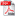# THEORETICAL RESEARCHES ON THE STRESS STATE AT VIBRO-DRAWING

Autor/autori: Octavian BOLOGA

Abstract: The paper contains the mathematical modelling of the stress state at vibro-drawing for the case of introducing the vibrating motion on axial direction, by means of the punch, into the drawing die. Starting from the definition of the total radial stress as sum of the radial stress for the transformation of the flange dimension and the stress due to the bending-unbending of the material on the die radius, there was emphasised the influence of introducing axial vibrations on the radial stress for the transformation of the blank's flange dimensions. The determined mathematical relations show a decrease of the stress when a dynamic motion is introduced into the drawing dieDOWNLOAD PDF